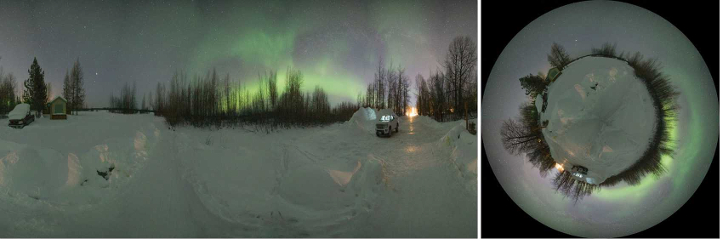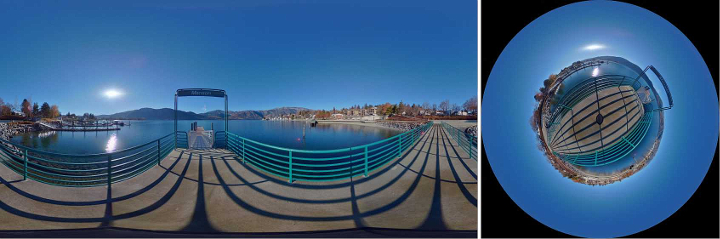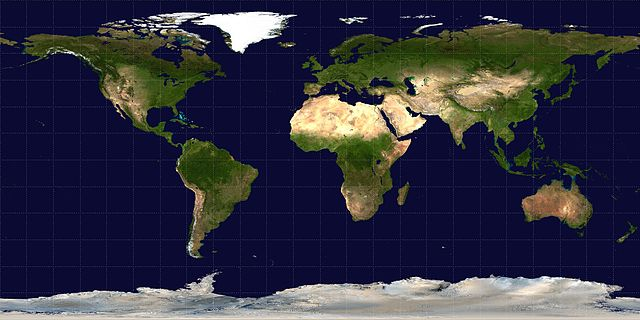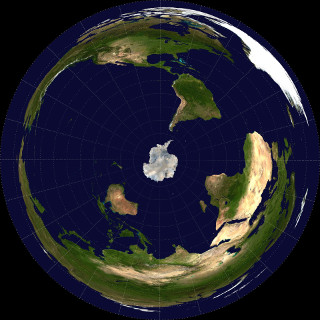# Equirectangular to Tiny-planet

Named Tiny planet, Little planet etc are beautiful effects that converts the projection of an equirecangular image.Source Image: Photo by Bryan GoffSource Image: Photo by Timothy Oldfield

This can be implemented using ffmpeg too:

Please use the latest ffmpeg version to try this. Latest static builds are also available here to try. I have used version ffmpeg version 4.4-static for these.

### Remapping Plugin

Ffmpeg got the useful remap plugin. It can remap any source pixels to destination pixels according to the input pixel mapping tables, or maps.

First we create the xmap and ymap required for the remap plugin.

The geq filter is used to create the maps we require

geq - apply generic equation to each pixel.

Full reference of the geq ffmpeg filter is here:

### Equations:

\begin{align} xMap_{(x, y)}=h\times\left(0.9999+\frac{atan2\left( y-h, x-h\right)}{\pi}\right) \\ yMap_{(x,y)} = h\times( {1-hypot((2\times{\frac{x}{w})-1},(2\times{\frac{y}{w})-1})}) \end{align}

note,

$hypot(x,y) = \sqrt{(x^2+y^2)}$

### Create Maps

For the example, the Equirectangular input frame has height $h=1920$ and width $w=3840$

• x-map

ffmpeg -f lavfi -i nullsrc=size=3840x3840 -vf format=pix_fmts=gray16le,geq="1920*(0.9999 + atan2(Y-1920\,X-1920)/PI)" -frames 1 -y xmap.pgm

• y-maps

ffmpeg -f lavfi -i nullsrc=size=3840x3840 -vf format=pix_fmts=gray16le,geq="1920*(1-hypot((2*X/3840)-1\,(2*Y/3840)-1))" -frames 1 -y ymap.pgm

### Apply maps and remap !

Lets try with an actual equirectangular world map from wikipedia here.

Input image:ffmpeg -i in.png -i xmap.pgm -i ymap.pgm -lavfi "format=pix_fmts=rgb24,remap" -q:v 2 -y out.png

Remapped image: